•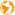网站地图
•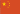中文版
•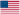English

HaiKe transformer

0755-27133533
136 9165 6577

Product Categories

0755-27133533
4产品中心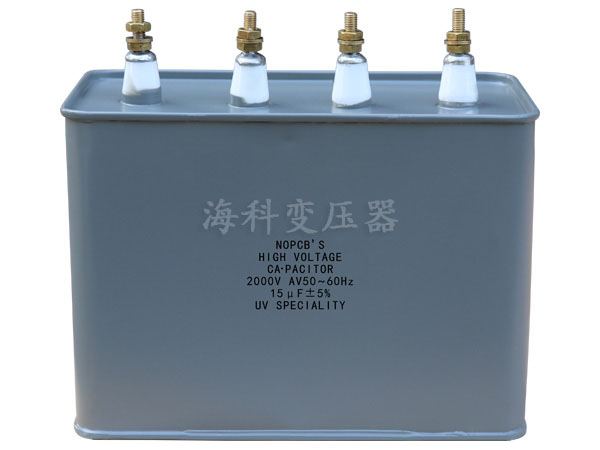UV电容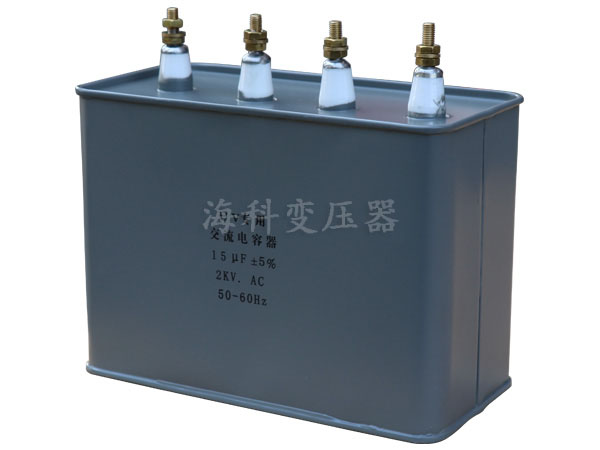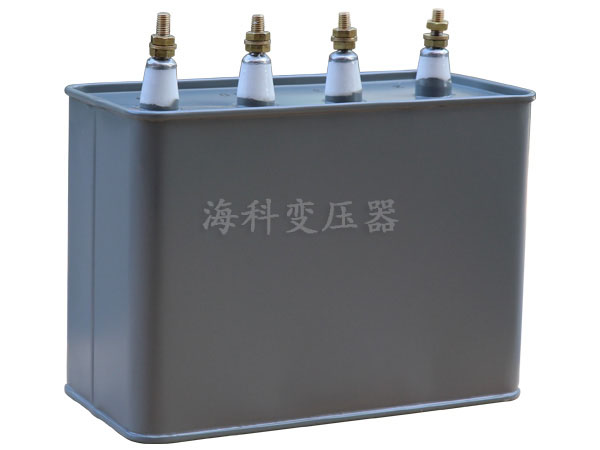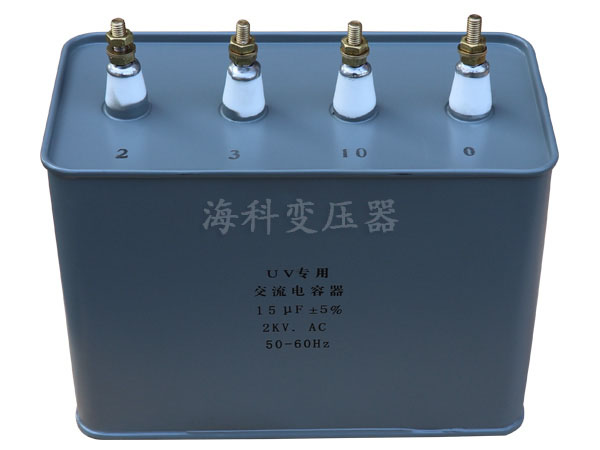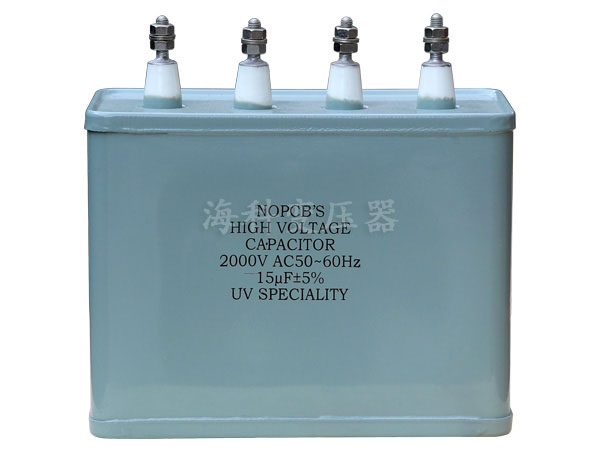#### UV电容

详情说明

UV电容器与漏磁变压器配套使用，UV电容器专门为特种光源、特种灯具研制生产的。在工作时补偿功率，使用时一般与变压器配套使用，作用在于补偿功率，平衡电流，使UV灯管保持在稳定的工作状态。其性能稳定，使灯保持在稳定的工作状态下正常工作。广泛用于高频、直流、交流和脉衡电路中。UV电容器是与UV变压器、UV灯管一起使用的光源类产品，该电容采用组合式，便于安装，制作功率范围广，并可根据用户的需求定做。

UV灯专用电容器，起补偿功率与平衡电流作用，是UV灯工作不可缺少的一部分。质量优良，价格合理，规格从1UF-300UF,耐压从1000V-5000V.广泛应用于高频、直流、交流和脉衡电路中。电容器与灯管、变压器是一起使用的光源类产品。采用组合式，便于安装，性能可靠防潮性好，寿命长等特点。

 额定电压kV~ 标称容量 μF 外壳编号 额定电压kV~ 标称容量 μF 外壳编号 HK-2 10+3+2 15 2 3.5 6+2+1.6 9.6 8 10+3+3 16 2 5+3+2 10 8 10+5+3 18 7 6+3+2 11 8 10+6+4 20 7 6+4+2 12 8 15+4+3 22 7 7+5+1 13 8 10+8+5 23 7 10+3+2 15 8 12+6+6 24 7 10+5+3 18 10 15+10+5 30 8 9+5+4 18 10 HK-2.5 6+3+3 12 8 4 2.2+1+1 4.2 8 8+4+3 15 8 2.2+1.4 4.6 8 10+3+2 15 8 3+1+1 5 8 8+7+1 16 8 3.3+2.2+1.1 6.6 8 8+8+2 18 8 5+3+1 9 8 10+5+3 18 8 5+3+2 10 8 15+3+2 20 8 5.7+1.7+1.4 8.8 8 HK-3 5+3+1 9 7 6+2+1.6 9.6 8 5+3+2 10 7 7+5+1 13 9 8+3+2 13 7 10+3+2 15 11 8+5+2 15 8 8+5+2 15 11 8+8+2 18 8 4.5 2+1.5+1 4.5 7 9+5+4 18 8 4+3+1.5 8.5 8 10+3+2 15 8 5+3+2 10 8 10+2+2 14 8 3+1+1 5 8 10+5+3 18 8 4+3+1.5 8.5 10 HK-3.5 5+2+1.5 8.5 8 5+2+1 8 10 5+3+1 9 8 10+3+2 15 11

•APP二维码
•公众号二维码